﻿ Dimensioning State Standard 2.307-68

# Dimensioning State Standard 2.307-68

Every drawing besides showing the true shape of an object, must supply its exact length, breadth, height, sizes and position of holes, grooves, etc. The procedure of presenting this information on a drawing sheet is called dimensioning. Lines, symbols, numerals and notes are used for this purpose.

There are two types of dimensions required to be shown on a drawing:

a) Overall dimension (size or functional dimension), and

b) Location or datum dimensions, shown by letters S and L respectively.

The size dimension indicates size such as length, breadth, height, diameter, etc.

The location dimension shows locations or exact position of various constructional details within the objects.

Fig. 1.8 shows the size and location dimensions.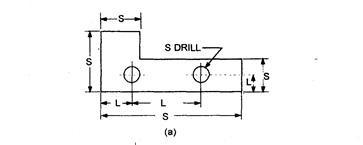Figure 1.8 – Size and location dimension

Extension and Dimension lines (continuous thin) are used for dimensioning (in mm)

The various types of dimension lines are:

1. Dimension line — It is a thin continuous line terminated by arrowheads touching the outlines or extension lines.

2. Extension line — It is a thin line drawn outside and along the outline.

3. Arrowhead — An arrowhead is placed at each end of a dimension line. Its pointed end touches an outline or an extension line. The length of arrowhead should be min 2.5mm. (fig.1.9).

Dimension lines are drawn parallel to a segment which is measured or on the concentric arc of measureable corner and limited the arrowheads (fig. 1.9).

It is desirable to draw dimension lines out of the contour of the depiction. It is necessary to avoid crossing of dimension and extension lines.

The lines of contour, axial, central and extension lines are not used as dimension lines.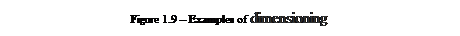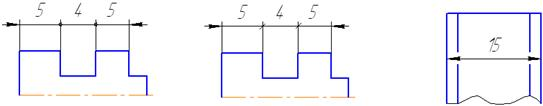It is allowed to replace pointers notch which are drawn under the corner of 45° to the dimension lines, or clear points if a place is not enough for arrowheads on dimension lines. If a place is not enough for arrowheads through the close located contour or extension line it can be cut (fig. 1.10).

Figure 1.10 Examples of dimensioning

The dimensions are placed above the dimension lines and may be read either from the bottom or from the right side of the drawing (fig. 1.11).

Linear dimensions on drawings are indicated in mm, and angular – in degrees, minutes, seconds with denotation of metages. Dimension number 5 mm high is marked above a size line in the distance of 0,5... 1 mm as possible nearer to the middle. Above parallel lines (or by concentric arcs) dimension numbers fill in offset (fig. 1.11 – a). Dimensioning at various inclinations of dimension lines is shown in figure 1.11 –b, c.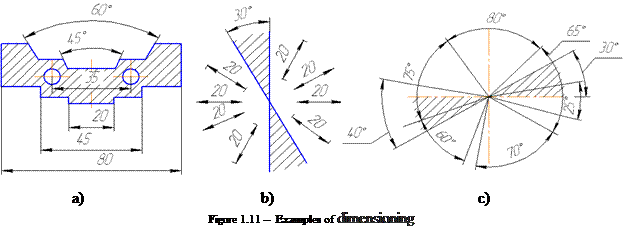Dimensions of diameters should be followed by symbol Æ before a dimension.

The circles should be dimensioned as shown in Fig. 1.12.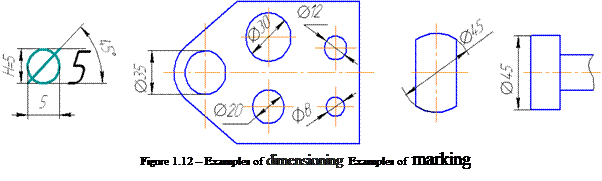The Latin letter R is put before a dimension while dimensioningof radius .

Some examples of dimensioning of radiuses are shown in figure 1.13.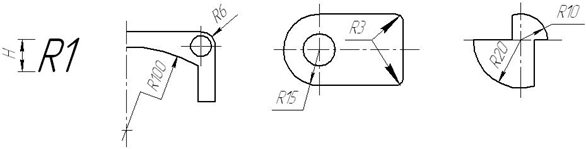Figure 1.13– Examples of dimensioning

Location of holes should be dimensioned where possible in the plan view of holes as shown in Fig. 1.14.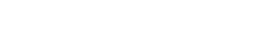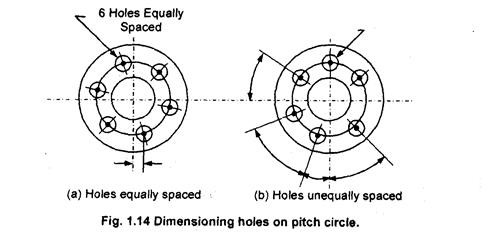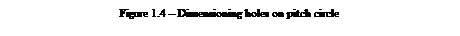A dimension line can be broken in cases, as shown in figure 1.15 a.

The square should be dimensioned as shown in 1.15 b.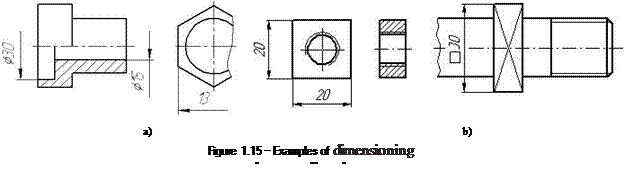As a rule, the size of the same elements of a product is applied once and representing the number of elements on a shelf or under a shelf extension line (figure 1.16 a).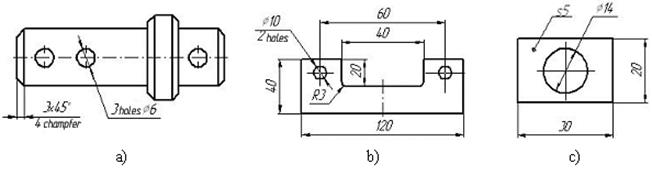Figure 1.16 - Examples of dimensioning.

The sizes of two symmetrically placed elements (radius R3) of the product (except of holes or chamfers) are marked one time without pointing their amount (fig.1.16 b). The dimension of thickness of a part in one projection (plank) are marked on a foot-note-shelf (s5) as shown in figure 1.16 c.

The size of a spherical surface should be dimensioned as shown in Fig. 1.17. A sphere is marked by the signs Æ or «R». If it is difficult to distinguish a sphere from other surfaces, the word «Sphere» is written or the sign Æ is put before a dimension.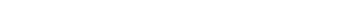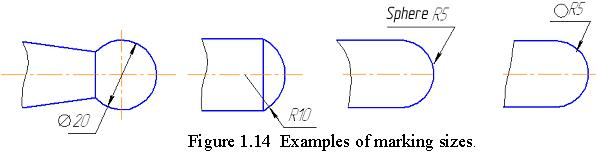Figure 1.17 - Examples of dimensioning

A chamfer is a beveled edge connecting two surfaces. If the surfaces are at right angles, the chamfer will typically be symmetrical at 45 degrees.

The chamfers are dimensioned by two linear sizes or by linear and angular sizes. The chamfers made under the angle of 45±, are marked through a sign “X”. Some examples of dimensioning the chamfers are shown in figure 1. 18.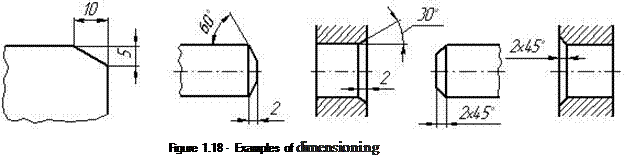There are two basic methods of dimensioning: progressive dimensioning and continuous dimensioning.

1. Progressive dimensioning — In this method of dimensioning, all dimensions on the drawing are shown from a common base line, as shown in Fig. 1.19. Cumulative error is avoided in this method.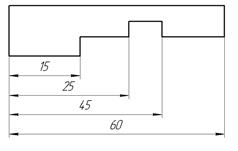Figure 1.19 - Progressive dimensioning

2. Continuous dimensioning — In this method, dimensions are arranged in a straight line. An overall dimension is placed outside the smaller dimension. One of the smaller dimensions is generally omitted which is least important, as shown in Fig. 1.20.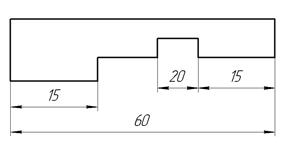Figure 1.20 - Continuous dimensioning

Finally, there are the general rules for dimensioning. The following rules should be observed while dimensioning:

1. Standard sizes of letters and figures should be used.

2. All dimensions should be specified in mm.

3. As far as possible dimensions should be placed outside the views.

4. Dimension lines should not run in the direction included in the hatched area.

5. Dimensions should be taken from visible outlines rather than from dashed lines.

6. Dimensions should be given from a base line, a centre line, an important hole, or a finished surface which may be readily established.

7. Dimensions should be quoted only once in one view.

8. Overall dimensions should be placed outside the intermediate dimensions.

9. Dimensions should be placed outside a sectional view.

10. Dimension line should not cross. Also dimension lines and extension lines should not cross.

11. When there are several dimension lines, the shorter dimension should be nearer the view.

12. Do not use outlines for dimensions.

Дата добавления: 2016-07-18; просмотров: 2247; ЗАКАЗАТЬ НАПИСАНИЕ РАБОТЫ

Поиск по сайту:

Воспользовавшись поиском можно найти нужную информацию на сайте.

Поделитесь с друзьями:

Считаете данную информацию полезной, тогда расскажите друзьям в соц. сетях.
﻿Poznayka.org - Познайка.Орг - 2016-2022 год. Материал предоставляется для ознакомительных и учебных целей.
Генерация страницы за: 0.036 сек.